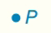Chapter 7.CT, Problem 5CT### Elementary Geometry for College St...

6th Edition
Daniel C. Alexander + 1 other
ISBN: 9781285195698

#### Solutions

Chapter
Section### Elementary Geometry for College St...

6th Edition
Daniel C. Alexander + 1 other
ISBN: 9781285195698
Textbook Problem
1 views

# Describe the locus of points in space that are at a distance of 3 cm from point P.___________________________________________________________________________________To determine

To describe:

The locus of points in space that are at a distance of 3 cm from point P.

Explanation

Definition:

A sphere (the three-dimensional object) is the locus of points in a space that are at a fixed distance from a given point.

The sphere has the fixed point as center, and the fixed distance determines the length of the radius.

Calculation:

A locus the point is the same distance away from the point at all points...

### Still sussing out bartleby?

Check out a sample textbook solution.

See a sample solution

#### The Solution to Your Study Problems

Bartleby provides explanations to thousands of textbook problems written by our experts, many with advanced degrees!

Get Started

#### Find the remaining trigonometric ratios. 29. sin=35,02

Single Variable Calculus: Early Transcendentals, Volume I

#### Evaluate the integrals in Problems 1-32. 12.

Mathematical Applications for the Management, Life, and Social Sciences

#### In Exercises 47-52, find and simplify f(a+h)f(a)h(h0) for each function. 52. f(x)=x

Applied Calculus for the Managerial, Life, and Social Sciences: A Brief Approach

#### Sometimes, Always, or Never: fxy = fyx.

Study Guide for Stewart's Multivariable Calculus, 8th